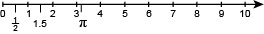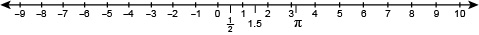Succeed with maths – Part 1

Start this free course now. Just create an account and sign in. Enrol and complete the course for a free statement of participation or digital badge if available.

Free course

# 1 Understanding negative numbers

It took mathematicians a long time to come to terms with negative numbers, so do not be surprised if you need to work slowly and carefully through this week’s study.

One thing that can really help you to understand more complex ideas, that arise in any university level study, are diagrams and negative numbers are no exception to this rule. So you are going to extend the work you did on number lines in Week 1 to help with this. Firstly, you'll have a quick reminder of how to use these.

You already know that positive whole numbers – known as natural numbers – are not sufficient to describe the world around us. Think about going to the supermarket and the various prices you see, most of these will have a decimal component showing the number of pence you have to pay. You also know that there are fraction and decimal numbers. One example of an irrational number that you came across earlier was π (pronounced pi) equal to about 3.14 – you can show all these numbers on a number line as illustrated in Figure 1.Figure _unit8.1.1 Figure 1 Positive numbers on a number line

What happens if you extend this number line to the left? What numbers are represented to this new section? These are the negative numbers, which are all less than zero. The number line now looks like this:Figure _unit8.1.2 Figure 2 Negative numbers on a number line

Can you think of a number line that you use, that has negative numbers on it? How about a thermometer? For example, on a cold winter morning, your thermometer might read ‘-3 °C’. This number is read as ‘minus 3 degrees Celsius’ and it means 3 degrees below zero. Using the number line example gives you a useful way to start exploring these new numbers, as you’ll see in the next section.

Before you do that though, look at the convention used to show negative numbers in the rest of this course.

Throughout your work, negative numbers will be denoted by using a small subtraction sign, i.e. ‘-3’. However, when negative numbers are used in calculations they will also be enclosed in brackets, to make it clear that they are negative numbers. This is a convention that you will see in some other maths text books and material but not all.

Now back to thermometers!